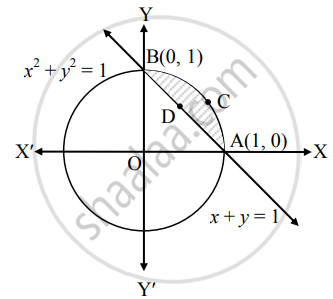# Find the area enclosed between the circle x2 + y2 = 1 and the line x + y = 1, lying in the first quadrant - Mathematics and Statistics

Sum

Find the area enclosed between the circle x2 + y2 = 1 and the line x + y = 1, lying in the first quadrant

#### Solution

Given equation of the circle is x2 + y2 = 1  ......(i)

and equation of the line is x + y = 1   ......(ii)

∴ y = 1 – x

From (i), we get

y2 = 1 – x2

∴ y = sqrt(1 - x^2)     ......(iii) ......[∵ In first quadrant, y > 0]

Substituting (ii) in (i), we get

x2 + (1 – x)2 = 1

∴ x2 + 1 – 2x + x2 = 1

∴ 2x2 – 2x = 0

∴ 2x(x – 1) = 0

∴ x = 0 or x = 1

When x = 0, y = 1 and when x = 1, y = 0Required area = area of the region ACBDA

= area of the region OACBO – area of the region OADBO

= area under the circle x2 + y2 = 1 – area under the line x + y = 1

= int_0^1 sqrt(1 - x^2)  "d"x - int_0^1 (1 - x)  "d"x   .....[From (iii) and (ii)]

= [x/2 sqrt(1 - x^2) + 1/2  sin^-1 (x)]_0^1 - [x - x^2/2]_0^1

= [0 + 1/2  sin^-1 (1) - 0] - [1 - 1/2 - 0]

= 1/2(pi/2) - 1/2

= (pi/2 - 1/2) sq.units

Concept: Area Between Two Curves
Is there an error in this question or solution?